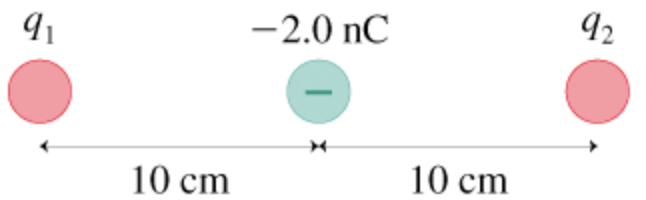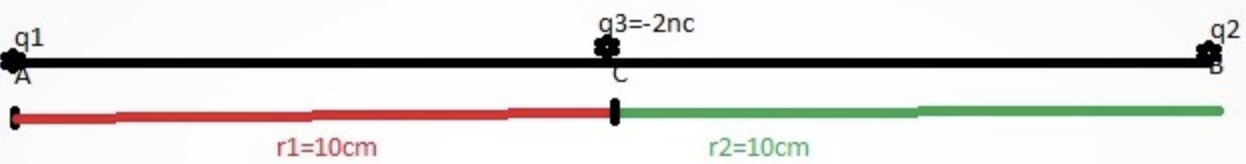In: Physics

# In (Figure 1), charge q2 experiences no net electric force. What is q1?

In (Figure 1), charge q2 experiences no net electric force. What is q1?## Solutions

##### Expert Solution

Concepts and reason

The concept required to solve this problem is electric force and Coulomb's law. Initially, obtain the expression for net electric force on $$q_{2}$$ by using expression for electric force and knowing direction of force due to charge $$q_{1}$$ and $$-2.0 \mathrm{n} \mathrm{C}$$ then find the charge $$q_{1}$$.

Fundamentals

According to Coulomb's law, the electric force between two charged particles is directly proportional to the product of their magnitude of charges and inversely proportional to the square of the distance between them. In equation form, Coulomb's law can be stated as $$F=\frac{k q_{1} q_{2}}{r^{2}}$$

Here, $$q_{1}$$ and $$q_{2}$$ are the charges separated by a distance $$r$$ and $$k$$ is the Coulomb's constant.

Use Coulomb’s law, to solve for the magnitude of the force of one charge on another. Also use the idea that charges of the same signs repel while charges of opposite signs attract.Distance between charges $$q_{1}$$ and $$q_{3}$$ is,

$$\begin{array}{c} r_{1}=10 \mathrm{~cm} \\ =10 \times 10^{-2} \mathrm{~m} \end{array}$$

Distance between charges $$q_{3}$$ and $$q_{2}$$ is,

$$\begin{array}{c} r_{2}=10 \mathrm{~cm} \\ =10 \times 10^{-2} \mathrm{~m} \end{array}$$

So distance between charges $$q_{1}$$ and $$q_{2}$$ is,

$$r=r_{1}+r_{2}$$

Substitute $$10 \times 10^{-2} \mathrm{~m}$$ for $$r_{1}$$ and $$r_{2}$$ in equation $$r=r_{1}+r_{2}$$ as follows:

$$\begin{array}{c} r=10 \times 10^{-2} \mathrm{~m}+10 \times 10^{-2} \mathrm{~m} \\ =20 \times 10^{-2} \mathrm{~m} \\ =0.2 \mathrm{~m} \end{array}$$

The net force on $$q_{2}$$ due to $$q_{1}$$ and $$q_{3}$$ is given by, $$F_{\text {neton } q 2}=F_{q 1 \text { on } q 2}+F_{q 3 \text { on } q 2}$$

Here, $$F_{q 3 \text { on } q 2}$$ is the force due to $$q_{3}$$ on $$q_{2}$$ and $$F_{q 1 \text { on } q 2}$$ is the force due to $$q_{1}$$ on $$q_{2}$$ The force on $$q_{2}$$ due to $$q_{1}$$ is $$F_{q 1 \mathrm{on} q 2}=\frac{k q_{1} g_{2}}{(r)^{2}}$$

Substitute $$0.2 \mathrm{~m}$$ for $$r$$ in equation $$F_{q 1 \mathrm{on} q 2}=\frac{k q_{1} q_{2}}{(r)^{2}}$$ as follows:

$$F_{q 1 \mathrm{on} q 2}=\frac{k q_{1} q_{2}}{(0.2 \mathrm{~m})^{2}}$$

The force on $$q_{2}$$ due to $$q_{3}$$ is $$F_{q 3 \text { on } q 2}=\frac{k q_{3} q_{2}}{\left(r_{2}\right)^{2}}$$

Substitute $$0.1 \mathrm{~m}$$ for $$r_{2}$$ and $$-2 \times 10^{-9} C$$ for $$q_{3}$$ in equation $$F_{q 3 \mathrm{on} q 2}=\frac{k q_{3} q_{2}}{\left(r_{2}\right)^{2}}$$ as follows:

$$F_{q 3 \mathrm{on} q 2}=\frac{k q_{2}\left(-2 \times 10^{-9} C\right)}{(0.2 \mathrm{~m})^{2}}$$

Substitute $$\frac{k q_{2}\left(-2 \times 10^{-9} C\right)}{(0.2 \mathrm{~m})^{2}}$$ for $$F_{q 3 \mathrm{on} q 2}$$ and $$\frac{k q_{1} q_{2}}{(0.2 \mathrm{~m})^{2}}$$ for $$F_{q_{1} \text { on } q 2}$$ in equation $$F_{\text {neton } q 2}=F_{q_{1} \text { on } q_{2}}+F_{q 3 \text { on } q 2}$$ as follows:

$$\begin{array}{l} F_{\text {neton } q_{2}}=\frac{k q_{1} q 2}{(0.2 \mathrm{~m})^{2}}+\frac{k\left(-2 \times 10^{-9} \mathrm{C}\right) q_{2}}{(0.10 \mathrm{~m})^{2}} \\ \quad=\frac{k q_{1} q_{2}}{(0.2 \mathrm{~m})^{2}}-\frac{k\left(2 \times 10^{-9} \mathrm{C}\right) q_{2}}{(0.10 \mathrm{~m})^{2}} \end{array}$$

Here assume that the charge $$q_{2}$$ is positive. Considering the forces on positive charge $$q_{2}$$. The other positive charge,

$$q_{1}$$, exerts a repulsive force $$q_{2}$$ that pushes $$q_{2}$$ away from $$q_{1}$$, that is, to the right. The negative charge $$q_{3}$$ exerts an

attractive force on $$q_{2}$$ that pulls $$q_{2}$$ toward $$q_{3}$$, that is, to the left. For the net force on $$q_{2}$$ to be zero, these two forces must have the same magnitude.

Equate the net force on $$q_{2}$$ to zero, $$F_{\text {neton } q_{2}}=0$$ $$k q_{2}\left[\frac{q 1}{(0.2 \mathrm{~m})^{2}}-\frac{2 \times 10^{-9} \mathrm{C}}{(0.10 \mathrm{~m})^{2}}\right]=0$$

Rearrange the above equation as follows:

$$\begin{array}{c} {\left[\frac{q_{1}}{(0.2 \mathrm{~m})^{2}}-\frac{2 \times 10^{-9} \mathrm{C}}{(0.10 \mathrm{~m})^{2}}\right]=0} \\ \frac{q_{1}}{(0.2 \mathrm{~m})^{2}}=\frac{2 \times 10^{-9} \mathrm{C}}{(0.10 \mathrm{~m})^{2}} \\ q_{1}=\frac{(0.2 \mathrm{~m})^{2} \times\left(2 \times 10^{-9} \mathrm{C}\right)}{(0.10 \mathrm{~m})^{2}} \\ =8 \times 10^{-9} \mathrm{C} \end{array}$$

Convert the units of charge from Coulomb's to Nano Coulombs as follows:

$$\begin{array}{c} q_{1}=8 \times 10^{-9} \mathrm{C}\left(\frac{1 \mathrm{nC}}{10^{-9} \mathrm{C}}\right) \\ =8 \mathrm{nC} \end{array}$$

Charge $$q_{1}$$ is $$8 \mathrm{nC}$$.

The Charge $$q_{1}$$ is $$8 \mathrm{nC}$$.

The charged particles are point charges. The charge $$q_{2}$$ is in static equilibrium, so the net force on $$q_{2}$$ is zero.

The Charge $$q_{1}$$ is $$8 \mathrm{nC}$$.